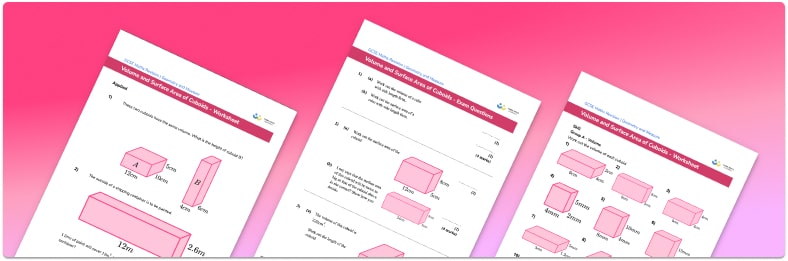# Volume And Surface Area Of A Cuboid WorksheetHelp your students prepare for their Maths GCSE with this free volume and surface area of cuboids worksheet of 44 questions and answers

• Section 1 of the volume and surface area of cuboids worksheet contains 36 skills-based volume and surface area of cuboids questions, in 3 groups to support differentiation
• Section 2 contains 4 applied volume and surface area of cuboids questions with a mix of word problems and deeper problem solving questions
• Section 3 contains 4 foundation and higher level GCSE exam style volume and surface area of cuboids questions
• Answers and a mark scheme for all volume and surface area of cuboids questions are provided
• Questions follow variation theory with plenty of opportunities for students to work independently at their own level
• All questions created by fully qualified expert secondary maths teachers
• Suitable for GCSE maths revision for AQA, OCR and Edexcel exam boards

• This field is for validation purposes and should be left unchanged.

You can unsubscribe at any time (each email we send will contain an easy way to unsubscribe). To find out more about how we use your data, see our privacy policy.

### Volume and surface area of cuboids at a glance

A cuboid (or rectangular prism) is a 3D shape made up of six rectangular faces. We can use different methods to calculate the volume of a cuboid and the surface area of a cuboid. A cube is a special cuboid where all of the side lengths are equal and all of the faces are squares.

To find the volume of a cube or cuboid we use the following formula: volume of a cuboid = length x width x height. This is another way of writing the formula for the volume of prisms, where width x height is the area of the cross-section of the cuboid, so the volume of a cuboid is the area of the cross-section x length.

To find the surface area of the cuboid we find the area of each face and add them together to calculate the total surface area. In order to find the total surface area of a cube we only need to find the area of one of the faces and then multiply it by 6 because each of the 6 faces of a cube is the same. Surface area is measured in units squared.

Looking forward, students can then progress to additional 3D shapes worksheets and more geometry worksheets, for example an angles in polygons worksheet or how to calculate volume worksheet.For more teaching and learning support on Geometry our GCSE maths lessons provide step by step support for all GCSE maths concepts.

## Do you have KS4 students who need more focused attention to succeed at GCSE?There will be students in your class who require individual attention to help them succeed in their maths GCSEs. In a class of 30, it’s not always easy to provide.

Help your students feel confident with exam-style questions and the strategies they’ll need to answer them correctly with our dedicated GCSE maths revision programme.

Lessons are selected to provide support where each student needs it most, and specially-trained GCSE maths tutors adapt the pitch and pace of each lesson. This ensures a personalised revision programme that raises grades and boosts confidence.

Find out more